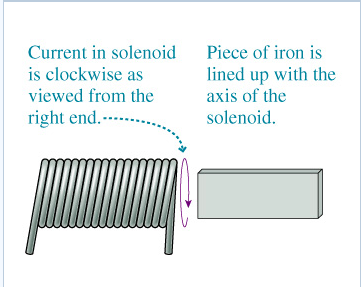# Problem: A solenoid is near a piece of iron, as shown in the figure. If a current is turned on in the solenoid, a magnetic field is created. This magnetic field will magnetize the iron, and there will be a net force between the solenoid and the iron. (Figure 1)Current in solenoid is clockwise as viewed from the Piece of iron is lined up with the axis of the solenoidA) Will the force on the iron be attractive or repulsive? (Choose one)B) Suppose this force moves the iron. Which way will the iron move?To the right away from the solenoidTo the left towards the solenoid(Choose one)C) As the iron moves, what will happen to the strength of the force?It will decreaseIt will increaseIt will not change(Choose one)

###### FREE Expert Solution

A)

After the iron is magnetized, the N-pole of the iron bar will be near the S-pole of the solenoid.

96% (302 ratings)###### Problem Details

A solenoid is near a piece of iron, as shown in the figure. If a current is turned on in the solenoid, a magnetic field is created. This magnetic field will magnetize the iron, and there will be a net force between the solenoid and the iron. (Figure 1)Current in solenoid is clockwise as viewed from the Piece of iron is lined up with the axis of the solenoid

A) Will the force on the iron be attractive or repulsive? (Choose one)

B) Suppose this force moves the iron. Which way will the iron move?

To the right away from the solenoid

To the left towards the solenoid

(Choose one)

C) As the iron moves, what will happen to the strength of the force?

It will decrease

It will increase

It will not change

(Choose one)

Frequently Asked Questions

What scientific concept do you need to know in order to solve this problem?

Our tutors have indicated that to solve this problem you will need to apply the Magnetic Field Produced by Loops and Solenoids concept. You can view video lessons to learn Magnetic Field Produced by Loops and Solenoids. Or if you need more Magnetic Field Produced by Loops and Solenoids practice, you can also practice Magnetic Field Produced by Loops and Solenoids practice problems.